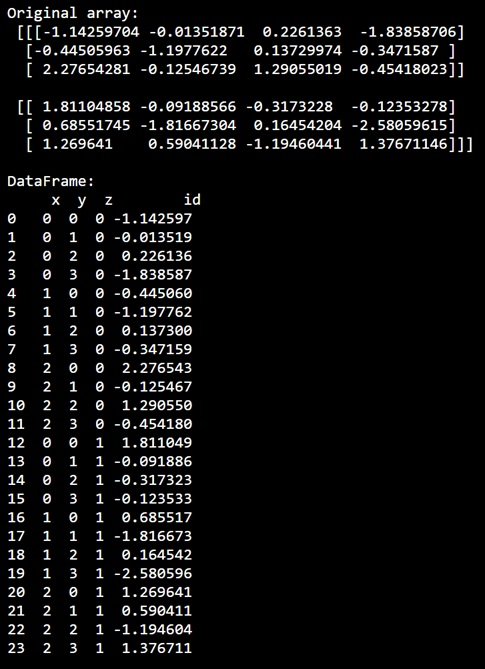# Converting a 3D NumPy array to coordinates and values

Learn, how to convert a 3D NumPy array to coordinates and values in Python?
Submitted by Pranit Sharma, on December 31, 2022

NumPy is an abbreviated form of Numerical Python. It is used for different types of scientific operations in python. Numpy is a vast library in python which is used for almost every kind of scientific or mathematical operation. It is itself an array which is a collection of various methods and functions for processing the arrays.

## Problem statement

Suppose we are given a 3D NumPy array that we want to convert into a DataFrame.

We need to convert it into a DataFrame so that the array elements become the coordinates and values.

## NumPy - Converting a 3D array to coordinates and values

For this purpose, we will first create an array and then we will define a dictionary of coordinates for which we will assign empty value lists. Finally, we will loop over a defined range to set up the coordinates and values of the DataFrame.

Let us understand with the help of an example,

## Python program to convert a 3D NumPy array to coordinates and values

```# Import numpy
import numpy as np

# Import pandas
import pandas as pd

# Creating a numpy array
arr = np.random.randn(2,3,4)

# Display original array
print("Original array:\n",arr,"\n")

# Creating a dictionary for mapping the values
d = {
'x': [],
'y': [],
'z': [],
'id': [],
}

# Looping for fixing the values
for z in range(2):
for x in range(3):
for y in range(4):
d['x'].append(x)
d['y'].append(y)
d['z'].append(z)
d['id'].append(arr[z][x][y])

# Creating dataframe
df = pd.DataFrame.from_dict(d)

# Display dataframe
print("DataFrame:\n",df,"\n")
```

### Output

The output of the above program is: